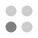• 5,877 trophies earned
• 295 Players Tracked
• 24 Total trophies
• 251 Obtainable EXP
• 1,200 Points
• 215 Platinum Club
• 215 100% Club

### Pic-a-Pix Classic Trophies

•Collect all the trophies72.88%
•Solve a 5x5 puzzle100.00%
•Solve a 10x10 puzzle98.64%
•Solve a 10x10 puzzle without using an X97.97%
•Solve all the 10x10 puzzles87.80%
•Earn a medal for every 10x10 puzzle86.10%
•Solve a 15x15 puzzle92.20%
•Solve a 15x15 puzzle without using an X91.53%
•Solve all the 15x15 puzzles77.29%
•Earn a medal for every 15x15 puzzle76.95%
•Solve a 20x15 puzzle86.78%
•Solve a 20x15 puzzle without using an X85.42%
•Solve all the 20x15 puzzles74.24%
•Earn a medal for every 20x15 puzzle73.90%
•Solve a 20x20 puzzle83.73%
•Solve a 20x20 puzzle without using an X83.05%
•Solve all the 20x20 puzzles75.93%
•Earn a medal for every 20x20 puzzle75.25%
•Solve a 30x20 puzzle85.08%
•Solve a 30x20 puzzle without using an X84.75%
•Solve all the 30x20 puzzles78.31%
•Earn a medal for every 30x20 puzzle77.29%
•Solve every puzzle73.56%
•Earn a medal for every puzzle73.22%